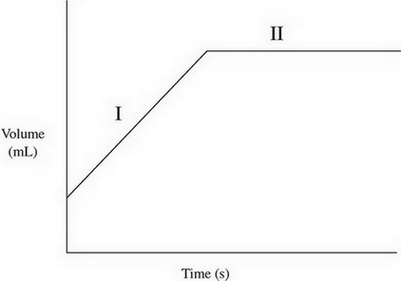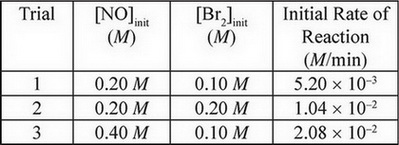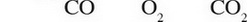# AP Chemistry Practice Test 6

### Test Information12 questions18 minutes

1. A compound is made up of entirely silicon and oxygen atoms. If there are 14.00 g of silicon and 32.0 g of oxygen present, what is the empirical formula of the compound?

2.The volume of a gas is charted over time, giving the above results. Which of the following options provides a possibel explinatin of what was happening to the gas during each phase of the graph?

3. A solution of sulfurous acid, H2SO3, is present in an aqueous solution. Which of the following represents the concentrations of three different ions in solution?

4. 2NO(g) + Br2(g) ↔ 2NOBr(g)

The above experiment was performed several times, and the following data was gathered:What is the rate law for this reaction?

5. SF4(g) + H2O(1) → SO2(g) + 4HF(g) ΔH = -828 kJ/mol

Which of the following statements accurately describes the above reaction?

`Questions 6-9 refer to the following information.20.0 mL of 1.0 M Na2CO3 is placed in a beaker and titrated with a solution of 1.0 M Ca(NO3)2, resulting in the creation of a precipitate. The conductivity of the solution is measured as the titration progresses.`

6. How much Ca(NO3)2 must be added to reach the equivalence point?

7. Which of the following diagrams correctly shows the species present in the solution in significant amounts at the equivalence point?

8. What will happen to the conductivity of the solution after additional Ca(NO3)2 is added past the equivalence point?

9. If the experiment were repeated and the Na2CO3 was diluted to 40.0 mL with distilled water prior to the titration, how would that affect the volume of Ca(NO3)2 needed to reach the equivalence point?

10. 2CO(g) + O2(g) → 2CO2(g)

2.0 mol of CO(g) and 2.0 mol of O2(g) are pumped into a rigid, evacuated 4.0-L container, where they react to form CO2(g). Which of the following values does NOT represent a potential set of concentrations for each gas at a given point during the reaction?11. Neutral atoms of chlorine are bombarded by high-energy photons, causing the ejection of electrons from the various filled subshells. Electrons originally from which subshell would have the highest velocity after being ejected?

12. A sample of oxygen gas at 50 °C is heated, reaching a final temperature of 100 °C. Which statement best describes the behavior of the gas molecules?